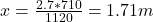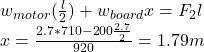## Two people carry a heavy electric motor by placing it on a light board 1.50 m long. One person lifts at one end with a force of 410 N, and t

Question

Two people carry a heavy electric motor by placing it on a light board 1.50 m long. One person lifts at one end with a force of 410 N, and the other lifts the opposite end with a force of 710 N.
a. What is the weight of the motor?
b. Where along with the board is its center of gravity located?
c. Suppose the board is not light but weighs 200, with its center of gravity at its center, and the two people each exert the same forces as before. What is the weight of the motor in this case?
d. Where is its center of gravity located?

in progress 0
1 month 2021-08-03T20:18:20+00:00 1 Answers 34 views 0

a) The weight of the motor is 1120 N

b) The distance of the center of gravity is 1.71 m

c) The weight of the motor is 920 N

d) The distance of the center of gravity is 1.79 m

Explanation:

a) The expression of the forces acting in the vertical direction is:

wmotor = F1 + F2

F1 = 410 N

F2 = 710 N

wmotor = 410 + 710 = 1120 N

b) The momentum of equilibrium about the center of the gravity is:

F1 * x = F2 * (l – x)

Clearing x:c) The expression for the forces acting in the vertical direction is:

wboard + wmotor = F1 + F2

wmotor = F1 + F2 – wboard

wmotor = 410 + 710 – 200

wmotor = 920 N

d) The same that b)